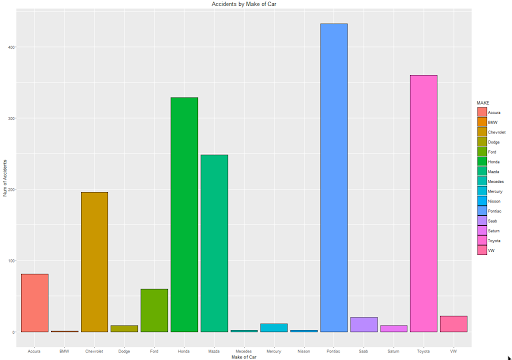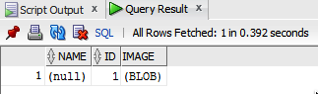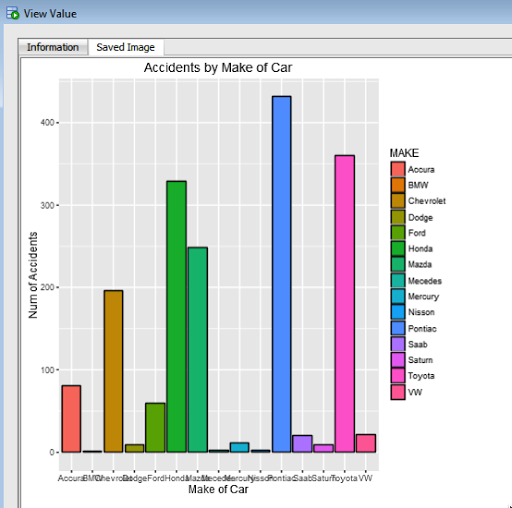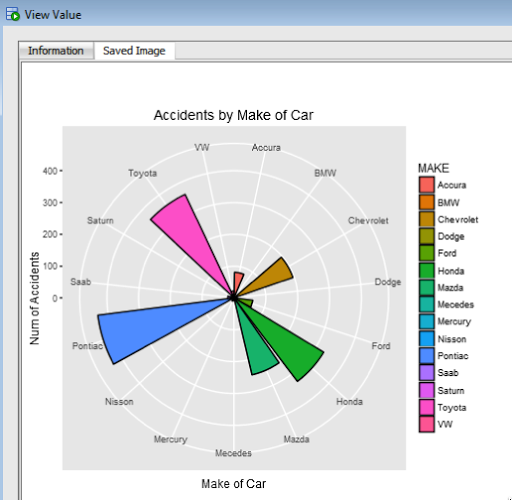### Creating ggplot2 graphics using SQL

Posted on

Did you read the title of this blog post! Read it again.

Yes, Yes, I know what you are saying, “SQL cannot produce graphics or charts and particularly not ggplot2 graphics”.

You are correct to a certain extent. SQL is rubbish a creating graphics (and I’m being polite).

But with Oracle R Enterprise you can now produce graphics on your data using the embedded R execution feature of Oracle R Enterprise using SQL. In this blog post I will show you how.

1. Pre-requisites

You need to have installed Oracle R Enterprise on your Oracle Database Server. Plus you need to install the ggplot2 R package.

In your R session you will need to setup a ORE connection to your Oracle schema.

2. Write and Test your R code to produce the graphic

It is always a good idea to write and test your R code before you go near using it in a user defined function.

For our (first) example we are going to create a bar chart using the ggplot2 R package. This is a basic example and the aim is to illustrate the steps you need to go through to call and produce this graphic using SQL.

The following code using the CLAIMS data set that is available with/for Oracle Advanced Analytics. The first step is to pull the data from the table in your Oracle schema to your R session. This is because ggplot2 cannot work with data referenced by an ore.frame object.

`data.subset <- ore.pull(CLAIMS) `

Next we need to aggregate the data. Here we are counting the number of records for each Make of car.

```aggdata2 <- aggregate(data.subset\$POLICYNUMBER,
by = list(MAKE = data.subset\$MAKE),
FUN = length)
```

Now load the ggplot2 R package and use it to build the bar chart.

```ggplot(data=aggdata2, aes(x=MAKE, y=x, fill=MAKE)) +
geom_bar(color="black", stat="identity") +
xlab("Make of Car") +
ylab("Num of Accidents") +
ggtitle("Accidents by Make of Car")
```

The following is the graphic that our call to ggplot2 produces in R.At this point we have written and tested our R code and know that it works.

3. Create a user defined R function and store it in the Oracle Database

Our next step in the process is to create an in-database user defined R function. This is were we store R code in our Oracle Database and make this available as an R function. To create the user defined R function we can use some PL/SQL to define it, and then take our R code (see above) and in it.

```BEGIN
-- sys.rqScriptDrop('demo_ggpplot');
sys.rqScriptCreate('demo_ggpplot',
'function(dat) {
library(ggplot2)

aggdata2 <- aggregate(dat\$POLICYNUMBER,
by = list(MAKE = dat\$MAKE),
FUN = length)

g <-ggplot(data=aggdata2, aes(x=MAKE, y=x, fill=MAKE)) + geom_bar(color="black", stat="identity") +
xlab("Make of Car") + ylab("Num of Accidents") + ggtitle("Accidents by Make of Car")

plot(g)
}');
END;
```

We have to make a small addition to our R code. We need need to include a call to the plot function so that the image can be returned as a BLOB object. If you do not do this then the SQL query in step 4 will return no rows.

4. Write the SQL to call it

To call our defined R function we will need to use one of the ORE SQL API functions. In the following example we are using the rqTableEval function. The first parameter for this function passes in the data to be processed. In our case this is the data from the CLAIMS table. The second parameter is set to null. The third parameter is set to the output format and in our case we want this to be PNG. The fourth parameter is the name of the user defined R function.

```select *
from table(rqTableEval( cursor(select * from claims),
null,
'PNG',
'demo_ggpplot'));
```

5. How to view the results

The SQL query in Step 4 above will return one row and this row will contain a column with a BLOB data type.The easiest way to view the graphic that is produced is to use SQL Developer. It has an inbuilt feature that allows you to display BLOB objects. All you need to do is to double click on the BLOB cell (under the column labeled IMAGE). A window will open called ‘View Value’. In this window click the ‘View As Image’ check box on the top right hand corner of the window. When you do the R ggplot2 graphic will be displayed.Yes the image is not 100% the same as the image produced in our R session. I will have another blog post that deals with this at a later date.

But, now you have written a SQL query, that calls R code to produce an R graphic (using ggplot2) of our data.

6. Now you can enhance the graphics (without changing your SQL)

What if you get bored with the bar chart and you want to change it to a different type of graphic? All you need to do is to change the relevant code in the user defined R function.

For example, if we want to change the graphic to a polar plot. The following is the PL/SQL code that re-defines the user defined R script.

```BEGIN
sys.rqScriptDrop('demo_ggpplot');
sys.rqScriptCreate('demo_ggpplot',
'function(dat) {
library(ggplot2)

aggdata2 <- aggregate(dat\$POLICYNUMBER,
by = list(MAKE = dat\$MAKE),
FUN = length)

n <- nrow(aggdata2)
degrees <- 360/n

aggdata2\$MAKE_ID <- 1:nrow(aggdata2)

g<- ggplot(data=aggdata2, aes(x=MAKE, y=x, fill=MAKE)) + geom_bar(color="black", stat="identity") +
xlab("Make of Car") + ylab("Num of Accidents") + ggtitle("Accidents by Make of Car") + coord_polar(theta="x")
plot(g)
}');
END;
```

We can use the exact same SQL query we defined in Step 4 above to call the next graphic.All done.

Now that was easy! Right?

I kind of is easy once you have been shown. There are a few challenges when working in-database user defined R functions and writing the SQL to call them. Most of the challenges are around the formatting of R code in the function and the syntax of the SQL statement to call it. With a bit of practice it does get easier.

7. Where/How can you use these graphics ?

Any application or program that can call and process a BLOB data type can display these images. For example, I’ve been able to include these graphics in applications developed in APEX.